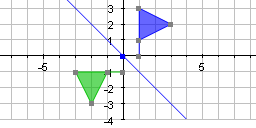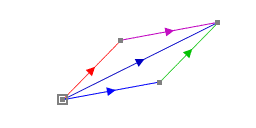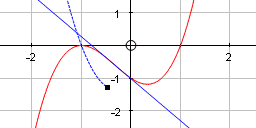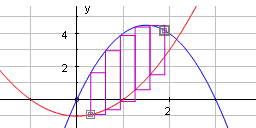## for the Dynamic Classroom

.. born in the classroom, and uniquely rich in pedagogy!
Ideal for graphing and geometry (2D and 3D),
statistics and probability.# 1D

Statistics & Probability# 2D

Graphing, coordinates, transformations and bi-variate data# 3D

Graphing, coordinates and transformations

###### Data Sets and Groupings

Data entered as either raw or grouped

• Continuous or discrete data

• Transform a data set

• Dynamicly change class width on grouped data

###### Generate data from probability distributions###### Representations of data
• Histograms including frequency density

• Cumulative Frequency diagrams

• Box and whisker diagrams

• Dot plots

• Mean and three standard deviations

• Line graph

• Moving average

• Stem and leaf

• Statistics box with set size, mean, standard deviation

• Table of statistics and tabulated values

###### Operations with data sets

Measurments of histograms and cumulative frequency diagrams Sample from data sets, demonstrating the central limit theorem###### Probability distributions

Plot and sample from

• Rectangular (discrete or continuous)

• Binomial

• Poisson

• Geometric

• Normal

• User defined, continuous or discreteMeasurments and hypothesis tests fitting to data/ fit dependent## 3rd Generation Graph Plotter for Schools and Colleges

###### 2D - Graphing

Creation of interactive objects based on movable points, lines, vectors, conics, polynomials, shapes, data sets.###### Shapes and transformations

Shapes either from a set of points or from a list of presets. Transformations including

• stretches

• shears

• reflections

• rotations

• enlargements

• translations

as well as arbitrary matrix transformations. Transformed objects update as the original object changes and can be chained. Rotations and enlargements can be animated, changing the value of the parameter, as can matrix transformations.###### Vectors

Create as an interactive object using points or with cartesian or polar coordinates. Vectors can be added, subtracted, multiplied, duplicated, have the angle between them found and can form the basis of straight lines and translations.

###### Equations

One line true notation entry in explicit, implicit, parametric, piece-wise, and polar forms.Slow plot allows you to draw curves incrementally, stopping at important points.

###### Calculus

Differentiate, find the area below a curve or between two curves. Integration treated as a first order differential equation.Differentiate, find the area below a curve or between two curves. Integration treated as a first order differential equation.###### Numerical methods

Bisection solution. Newton Raphson method. Step forwards and backwards.Numerical integration. Rectangle, trapesium and Simpson's methods. Animated the number of divisions.

###### Tangents and normals

Plot tangents and normals to curves; evolute draws these in many places along the curve, often leading to striking shapes.

###### Scatter graphs
• Data sets from points, files or entered through Autograph's spreadsheet

• Least squares regression graphically illustrated

• Fit straight lines, polynomial or fit your own equation to the data###### Points as the basis of objects

Form objects including:

• shapes

• lines

• vectors

• planes

###### Shapes

Either form shapes from joined triangles or select from a list of preset shapesTransform shapes:

• Rotations

• Reflections

• Enlargements

• Translations

as well as arbitrary matrix transformations.

###### Lines and planes
• Lines and planes from points, vectors or equations. Intersection of:

• Lines and planes

• Two planes

• Three planes###### Vectors

Vectors created from points or entered in Cartesian form. Add, subtract, copy, vector and scalar products.

###### Equations

Explicit, implicit, parametric, spherical and cylindrical polarsDraw normals and tangets to surfaces and parametric curves Acceleration and velocity vectors to parametric curves